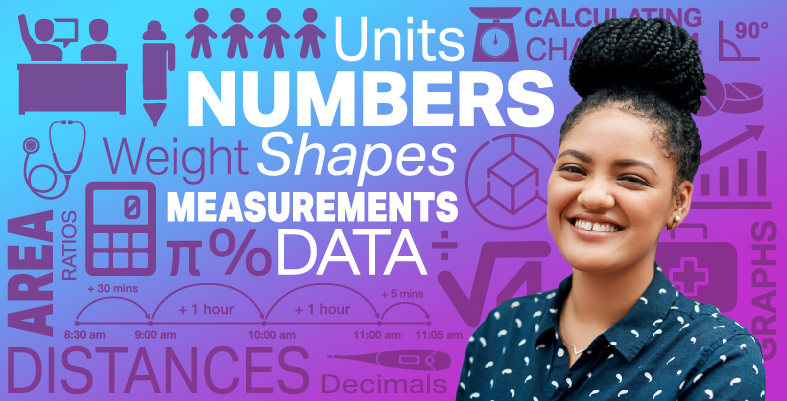Everyday maths for Health and Social Care and Education Support 2

Start this free course now. Just create an account and sign in. Enrol and complete the course for a free statement of participation or digital badge if available.

Free course

# 1 Units of measure

A unit of measure is simply what you measure something in and you will already be familiar with using centimetres, metres, kilograms, grams, litres and millilitres for measurement. When measuring something, you need to choose the appropriate unit of measure for the item you are measuring. You would not, for example, measure the length of a room in millimetres. Have a go at the short activity below and choose the most appropriate unit for each example.

## Activity 1: Choosing the unit

Using the following two lists, match each numbered item with the correct letter.

1. Millilitres (ml)

2. Metres (m)

3. Kilograms (kg)

4. Grams (g)

5. Centimetres (cm)

6. Litres (l)

• a.Length of a garden fence

• b.Weight of an egg

• c.Amount of water in a paddling pool

• d.Amount of liquid in a glass

• e.Length of a computer screen

• f.Weight of a dog

• 1 = d
• 2 = a
• 3 = f
• 4 = b
• 5 = e
• 6 = c

Hopefully you found that activity fairly straightforward. Next you’ll take a closer look at some units of measure and how to convert between them.

FSM_SSH_2